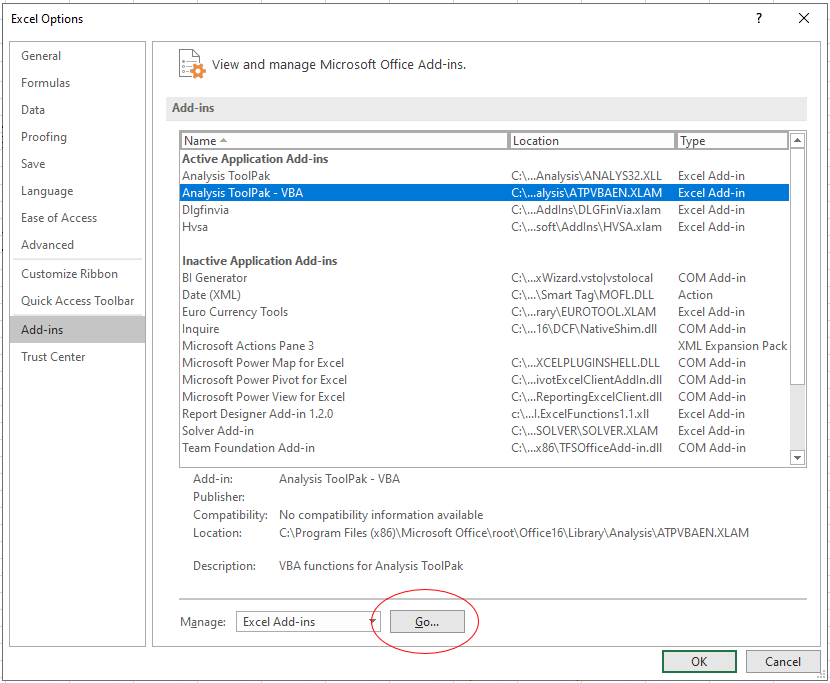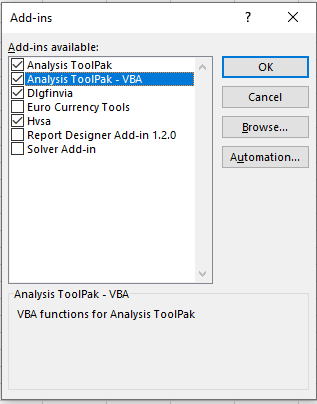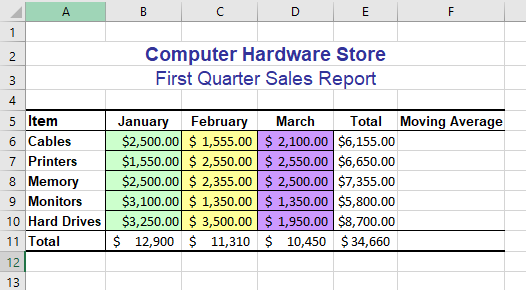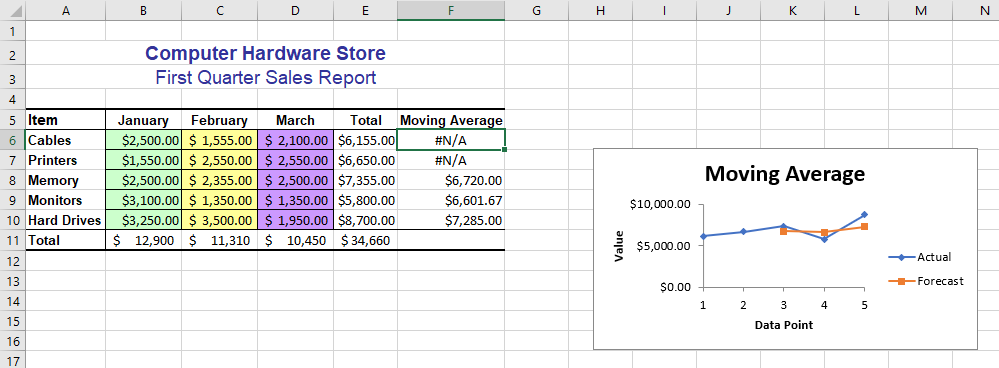# VBA – Using the Analysis ToolPak in VBA

This tutorial will show you how to use the Analysis ToolPak in VBA.

The Analysis ToolPak in Excel is an add-in created to enable you to do complicated Data Analysis.   If you wish to call these functions in VBA code, you need to enable the Analysis ToolPak-VBA Add-in.Click on the Go button.Make sure the Analysis ToolPak-VBA is ticked and click OK.

You will now be able to call the Functions contained in the Excel Analysis ToolPak from VBA.

## Using the Moving Average in VBA

Consider the following worksheet.To use a function from the add-in, you need to use Application.Run and then the name of the function you wish to call.

To calculate the moving average in column F, you can write the following code.

``````Sub CalculateMovingAverage()
Dim rngInput As Range
Dim rngOutPut As Range
'populate the ranges
Set rngInput = Range("E6:E10")
Set rngOutPut = Range("F6:F10")
'call the function from the add in
Application.Run "Moveavg", rngInput, rngOutPut, , False, True, False
End Sub``````If you do not want to output a chart, amend the Application.Run line to this

`` Application.Run "Moveavg", rngInput, rngOutPut, , False, False, False``

Stop searching for VBA code online. Learn more about AutoMacro - A VBA Code Builder that allows beginners to code procedures from scratch with minimal coding knowledge and with many time-saving features for all users!